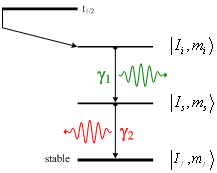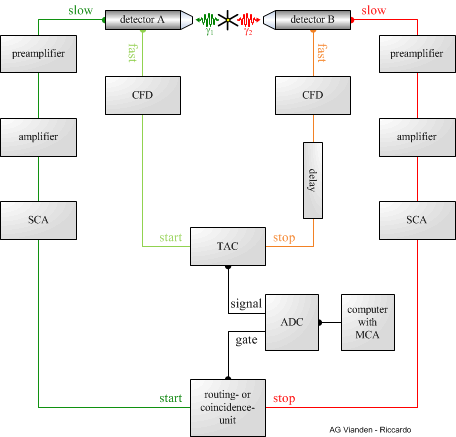# The perturbed angular correlation method

The technique of the perturbed angular correlation (PAC) belongs to the class of hyperfine spectroscopic methods as the Mößbauer-spectroscopy. The hyperfine interaction between the electromagnetic moments of the nuclear state and the external electromagnetic fields at nuclear site is measured. This technique can be used for determine the magnetic dipole and the electric quadrupole moments of the nuclear states and using nuclei with known moments for deriving the internal electromagnetic fields in solids, liquids, gases, ions and free atoms.

So the perturbed angular correlation started with being a technique used in nuclear physics, measuring spins and parities. With the development of the experimental techniques the angular correlation was recognised having a high potential in condensed matter research and today its applications are predominantly in this area.

The principle of perturbed angular correlation consists in producing a radioactive nuclei that decays over a γγ-cascade in the ground state of the daughter nucleus and to bring it into the host material under research. The initial state with nuclear spin |Ii, mi〉 decays (see figure 1) through the emission of a γ-quant γ1 in an interstitial state |Is, ms〉 and this under emission of a second γ-quant γ2 in the final state or ground state |If, mf〉.The emission of the first γ is isotropic. Detecting γ1 in a fixed direction k1 an ensemble of nuclei with oriented spins is selected. This produces an unequal (inhomogeneous) population of the m-states of the intermediate state. The detecting of the succeeding radiation in the direction of k2 is not isotropic anymore and shows a definite angular correlation with respect to the first.

The aim is to find the relative probability W(ϑ)dΩ of detecting γ2 in the solid angle dΩ with the angle ϑ in respect to γ1. This probability is given by the angular correlation function

 W(ϑ) = kmax∑ k AkkPk(cosϑ)
(1)

For a γγ-cascade is k even because of the conservation of parity and 0 < k < min(2Is, li+l′i). Here is Is the spin of the interstitial state and li with i=1;2 the multipolarities of the two transitions. For pure multipole transitions li=l′i. The parameters Akk are the so called angular correlation coefficients and are depending of the angular momenta of the state and the multipolarities of the transitions.

The radioactive nuclei, decaying through a succession of two γ-radiations, are often built in materials that have to be investigated. Here the probe nucleus can be perturbed during the finite lifetime of the intermediate state from the hyperfine interaction of magnetic or electric surroundings. Through this perturbation the angular correlation function is changing to

 W(ϑ) = kmax∑ k AkkGkk
(2)

where Gkk is the perturbation factor containing the information about the interaction. Both the magnetic and the electric interaction exercise a torque on the angular momentum of the intermediate state which produces a precession of Is around the symmetry axis. Quantum mechanically this means that the interaction can lead to transitions among the m-states. So the second radiation is emitted from a level with an altered population distribution and this change is responsible for the attenuation of the correlation. The interaction can occur between the nuclear magnetic dipole moment μ of the intermediate state Is and the extranuclear magnetic field B or between the nuclear electric quadrupole moment Q and the extranuclear electric field gradient V = ∇2Φ.

## Magnetic hyperfine interactions

For magnetic hyperfine interactions the frequency of the precession of the nuclear spin around the axis of the magnetic field B is the Larmor-frequency

 ωL = − g μN B ħ
(3)

where g is the g-factor (Landé-factor) of the intermediate state and μN the nuclear magneton (μN=5.05·10−24 [(erg)/(G)]=5.05·10−27 [(J)/(T)])1.

The precession involve a rotation of the intensity distribution of the γγ-cascade and the coincidence counting rate is subject to a modulation. Quantum mechanically the magnetic hyperfine interaction splits the intermediate state into |2I+1| equidistant sublevels. The energy difference between the sublevels is

 ∆E = ħ ωL = −g μN B
(4)

From the measurement of the Larmor-frequency it is possible to determine either the g-factor or the magnetic field, depending on which quantity is known.

## Static electric hyperfine interactions

Historically the technique of the perturbed angular correlation was used to measure the magnetic moments of excited nuclei and less for determine the electric quadrupole moments. The problem hereby was, that also with the strongest external electric field gradients the quadrupole hyperfine interaction was too small to be measured with known methods. Electric fiel gradient that are strong enough to be measured are found in non-cubic solids and non complete electron shells.

The energy of the electric hyperfine interaction between the charge distribution of the nucleus and the extranuclear static electric field can be expanded into mutlipoles. The monopole term solely causes an energy shift and the dipole term vanishes, so the first relevant expansion term is the quadrupole term

 EQ = ∑ ij QijVij        i,j = 1;2;3
(5)

which can be written as the product of the quadrupole moment Qij and the electric field gradient Vij. Both are tensors of the second order. Higher term of the expansion are too small to be detected with PAC.

The electric field gradient is the second derivate of the electric potential at nuclear site

 Vij = ∂2φ(0) ∂xi ∂xj
(6)

Through adequate principle axis representation it is possible to diagonalise Vij so that

 |Vzz| ≥ | Vyy| ≥ |Vxx|

Because of the Laplace equation, ∑ijVij=0, only two of the three components Vii are linear independent. Usually the largest component Vzz and the asymmetry parameter η, defined as

 η: = Vyy − Vxx Vzz
(7)

are chosen to characterise the electric field gradient.

For cubic crystals, which are equivalent in all directions x, y and z (therefore Vzz = Vyy = Vxx) and also for axially symmetric crystals, which are equivalent in x and y (therefore Vyy = Vxx) the asymmetry parameter vanishes η = 0. In cases of smaller symmetry η takes values between zero and one.

For axially symmetric electric field gradients the energy of the sublevels takes the values

 EQ = e Q Vzz 4I(2I−1) ·( 3m2 − I(I+1) )
(8)

The energy difference between two sublevels m and m′ is given by

 ∆EQ = Em − Em′ = e Q Vzz 4I(2I−1) ·3 |m2 − m′2|
(9)

 ωQ : = e Q Vzz 4I(2I−1) ħ
(10)

for the energy difference can be written

 ∆EQ = ħ ωQ ·3 |m2 − m′2|
(11)

In contrast to the magnetic interaction the splitting of the sublevels of the nuclear state through the electric quadrupole interaction depends on the angular moment I of the sublevel and is therefore non-equidistant. The transitions frequencies ωn between the different sublevels are, in case of η = 0, integer multiples of the smallest frequency ω0. It can be written

 ωn = n ·ω0
where
 ω0 : = min ⎛⎝ 1 ħ ·∆EQ ⎞⎠

whereas ω0 = 3 · ωQ for integer nuclear spin I and ω0 = 6 · ωQ for half integer nuclear spin I.

As for the magnetic dipole interaction, also the electric quadrupole interaction induces a precession of the angular correlation in time and this modulates the quadrupole interaction frequency. This frequency is an overlapping of the different transition frequencies ωn. The relative amplitudes of the different components depend on the orientation of the electric field gradient relative to the detectors (symmetry axis) and on the asymmetry parameter η. For a study with different nuclei one needs a parameter that allows a direct comparison, therefore the interaction frequency νQ is introduced, which is independent from the nuclear spin I.

 νQ : = e Q Vzz h
(12)

## Experimental setup

There are two different ways of measuring the angular correlation, the time integral and the time differential mode. The integral perturbed angular correlation (IPAC) is used if the lifetime of the intermediate state is smaller or similar to the experimental time resolution or for measuring strong internal magnetic fields of ferro magnets. The time differential perturbed angular correlation (TDPAC) measures the angular correlation as fraction of the time the nucleus spends in the intermediate state.Experimentally the number of coincident events between γ1 and γ2 is measured as a function of time. In a simple experimental setup (as shown in figure 2) the probe is put between two scintillation detectors which are connected to a fast-slow-coincidence circuit, so two signals are derived from each detector: the fast timing signal and the slow energy pulse. The fast timing signal is fed into the time-to-amplitude converter (TAC), an electronic clock, which allows to measure the time the nucleus spent in the intermediate state of the cascade. The TAC is started by the fast signal of the first detector and stopped by that of the second detector. Its output amplitude is than proportional to the time between the two signals-pulses. To assure that the TAC is started by the signal of γ1 and stopped by that of γ2 the slow output of the detectors, which is proportional to the energy of the incoming and detected radiation, is used. The right energy is chosen by setting the windows of the single channel analysers (SCA) whose output gates the multichannel analyser (MCA). This electronic device sorts the incoming signals by their amplitude in channels. Usually setups with more than two detectors are chosen so that the coincident counting rate increases and the time for achieving a reasonable statistic decreases. In the literature setups using up to eight detectors are reported; here a setup consisting of four detectors is used.

Starting from the equation 1 the number of coincidences measured by the apparatus can be calculated as

 N(ϑ,t)
 =
 N0 e−λt ·W(ϑ)
 N(ϑ,t)
 =
 N0 e−λt ·(1 + A22P2(cosϑ) + A44P4(cosϑ) )

where N0 is the total counting rate, λ the decay constant and the rest term describes the anisotropic emission probability. If the coefficient A44 is much smaller than A22 (A44 << A22) it is sufficient to determine the angular correlation at two fixed angles ϑ. Usually one uses the angles 90° and 180° because for this angles the Legendre polynomials assume extremely simple values (P2(cos90°)=−[1/2] and P2(cos180°)=1). Now to eliminate the exponential decay, which does not contain any information about the modulation, the ratio

 R(t) : = 2 · N(180°,t) − N(90°,t) N(180°,t) + 2N(90°,t)
(13)

is defined. Supposed the decay is not perturbed by external magnetic or electric fields, then the ratio R(t) is constant in time and equal to the coefficient A22.

### Footnotes:

1erg=10−7 J and 1 G=10−4 T (G = Gauss)

### Other sites with illustrations to PAC...

Here some useful links to sites, where you can find more or less detailed explanations about the PAC method.